# CBSE Class 9 Mathematics Quadrilaterals VBQs

Question.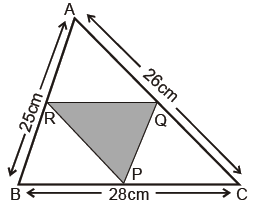There is a visit in a school by Directorate of Education. Girls are asked to prepare Rangoli in triangular shape. Dimensions of Δ ABC are 26 cm, 28 cm, 25 cm. Garland is to be placed along the side of ΔPQR which is formed by joining midpoints of sides of ΔABC.

(a) Find the length of garland.

(b) What values are depicted here ?

Answer: (a) RQ | | BC and RQ =1/2  BC

(Mid pt. theorem)

=RQ = 14 cm

QP = 12.5 cm

PR = 13 cm

Perimetre = 14+12.5+13

= 39.5 cm

(b) Beauty, happiness, co-operation

Question. A class teacher gave students coloured papers in the shape of quadrilateral. She asked them to make parallelogram from it using paper folding.

(a) How can a parallelogram be formed by using paper folding ?

(b) Prove that it is a parallelogram.

(c) What values are depicted here ?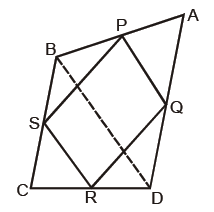(a) By joining mid pts. of sides of a quadrilateral.

(b) In ΔABD

P & Q are mid point of AB and AD

there for , PQ || BD & PQ 1/2 BD                ......................(i) (Mid point theorem)

similarly, RS || BD & RS 1 BD                     .......(ii)

from (i) & (ii) PQRS is a parallelogram

(c) Curiosity, Happiness, Scientific Temper

Question. ABCD is a rhombus where ΔADC=120°. There are two fire stations at C and D and fire is reported at O.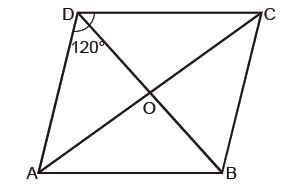(a) Which fire station team can reach early and why ?

(b) Which value is depicted here ?

Answer: (a) D will send the team early ODC = 60° & OCD = 30° Ð OC > OD (side opp. to larger angle is longer)

(b) Serving the people with honesty

Question. Sohan wants to show gratitude towards his teacher by giving her a cardmade by him. He has three pieces of trapezium pasted one above the other as shown in the fig. These pieces are arranged in a way that AB || HC || GD || FE. Also BC=CD=DE and GF=6 cm. He wants to decorate the card by putting up a coloured tape on non parallel sides of trapezium.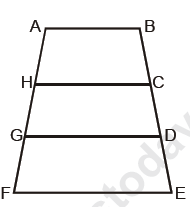(a) Find the total length of coloured tape required if DE = 4cm.

(b) Which value is depicted by the student ?

Answer: (a) AB || HC || GD || FE and BC = CD = DE

= AH = HG = GF

(given three parallel lines making equal intercepts on any transversal then they will make equal intercepts on other transversal also)

threre for, AF+FE = 18+12 = 30 cm

(b) Happiness, beauty, knowledge

Question. A farmer has a field in the form of a parallelogramABCD. One of his cow is suffering from some disease. To take good care of her, he tied the cow at one corner of the field. The corner angle of the field is 65°.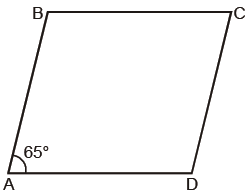(a) Find all the other three angles of the field.

(b) Explain the values depicted here.

Answer: (a) ÐA =ÐC = 65°ÐB = 180° – 65° =115° = ÐD

(b) Helpfulness, caring for every one

Question. A chocolate is in the form of a quadrilateral with sides 6 cm, 10 cm, 5 cm, 5 cm is cut into two parts along one of its diagonals by a lady. Part I is given to her maid and part II is equally divided among her driver and maali.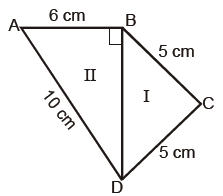(a) Is this distribution fair ? Justify it.

(b) What value is depicted here ?

(b) BD √ AD² - AB2 = √100 - 36 = √64

BD = 8 cm

ar (ABD)  =1/2* 6* 8

= 24 cm2

ar (BCD)

S=a+b+c/2 5+5+8/2 = 9 cm

area = √9* (9 - 5)*(9 - 5)*(9 -8)

=√ 9 *4* 4*1

= 12 cm2

Question. While analysing properties of quadrilateral a child says that all angles of a quadrilateral can not be obtuse.

(b) What value is depicted in this activity ?

Answer: (a) IfÐ A=91°, ÐB=91°, ÐC=91° then ÐD < 90°
as ÐA+ÐB+ÐC+ÐD = 360°

(b) Scientific temper, knowledge

Question. During Maths Lab Activity each student was given four broom sticks of lengths 8 cm, 8 cm, 5 cm, 5 cm to make different types of quadrilaterals.

(a) How many quadrilaterals can be formed using these sticks.

(b) Name the types of quadrilaterals formed.

(c) While doing this activity which value is depicted ?

(b) Rectangle, parallelogram, kite

(c) Scientific temper, curiosity

Question. In a trapezium ABCD   DC || AB and ÐA=ÐB=45°. teacher asked the student to find ÐD.Naresh answered it as 135°

(a) Is the answer correct ? Justify it.

(b) Which value is depicted here ?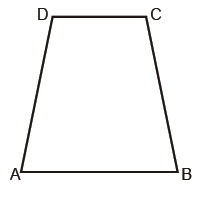Answer: (a) DC | | AB

=ÐA +ÐD = 180°

=ÐD = 180° – 45° = 135°

(b) Scientific temper, truth value

Question. While discussing the properties of a parallelogram teacher asked about the relation between two angles x and y of a parallelogram as shown in the fig. Teacher gave them three options as :(i) x > y (ii) x < y (iii) x = y

Beena gave the answer as x < y

(a) Is this the correct answer ?

(b) Justify the answer with suitable logic.

(c) What is the value depicted in this ?

(b) ÐADB =ÐDBC = y (alternate int. angles)

since BC < CD (angle opp. to smaller side is smaller)

there for, x < y

(c) Truth value

 CBSE Class 9 Mathematics Circles VBQs
 CBSE Class 9 Mathematics Construction VBQs
 CBSE Class 9 Mathematics Surface Areas And Volume VBQs
 CBSE Class 9 Mathematics Statistics VBQs
 CBSE Class 9 Mathematics Probability VBQs
 CBSE Class 9 Mathematics Linear Equations VBQs
 CBSE Class 9 Mathematics Quadrilaterals VBQs
 CBSE Class 9 Mathematics Areas Of Parallelograms And Triangles VBQs
 CBSE Class 9 Mathematics Value Based Questions

Tags